# Concept of equality conditional to existence of one side

## General concept

There are a number of theorems in mathematics which are of the following form:$\mbox{Left side expression} = \mbox{Right side expression}$

with a conditionality caveat as follows:

• Whenever the right side expression makes sense, the left side expression also makes sense and they are equal.
• It may, however, happen that the right side expression does not make sense but the left side expression does.

This concept occurs in theorems about limits and derivatives of pointwise combinations (sums, differences, products, quotients), as well as composites.

## More on the mechanics of how we get results like this

### Example of differentiation being additive

Consider the statement that differentiation is linear; one component of this statement is the assertion that differentiation is additive, i.e., that:$(f + g)'(x) = f'(x) + g'(x)$

This is an example of a statement that is true whenever the right side is defined; however, it is possible for the left side to be defined at a point and the right side to not be defined at that point.

For instance, consider the function$f(x) := x^{2/3}$ and$g(x) := -x^{2/3}$.

•$f$ is differentiable for all$x \ne 0$, with derivative$f'(x) = \frac{2}{3}x^{-1/3}$. At 0, the derivative is undefined, with a vertical cusp.
•$g$ is differentiable for all$x \ne 0$, with derivative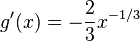$g'(x) = -\frac{2}{3}x^{-1/3}$. At 0, the derivative is undefined, with a vertical cusp.
•$f + g$ is identically the zero function, so its derivative is also$0$ everywhere.

We can see based on the above, that for all$x \ne 0$:$(f + g)'(x) = f'(x) + g'(x)$

However, at$x = 0$,$(f + g)'(x)$ is defined, whereas$f'(x) + g'(x)$ is not defined.

So we see in this case that the equality holds whenever the right side is defined, but the left side can be defined at some points where the right side isn't defined, and in those cases the equality fails to hold.

### Similar examples for product rule and chain rule

Similar examples can be obtained for the product rule for differentiation and the chain rule for differentiation:

• For the product rule for differentiation, consider$f(x) := x^{1/3}$ and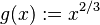$g(x) := x^{2/3}$. Neither is differentiable at$x = 0$, so the product rule for differentiation cannot be used at 0. However, the product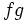$fg$ is the function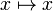$x \mapsto x$, which is differentiable everywhere,
• For the chain rule for differentiation, consider$f(x) := x^3$ and$g(x) := x^{1/3}$. The latter is not differentiable at$x = 0$, so the chain rule for differentiation cannot be used at 0. However, the composite$f \circ g$ is the function$x \mapsto x$, which is differentiable everywhere.

### What is going on? Cancellation, accumulation, and restitution

There are a few different ways of thinking about what is going on with the above examples.

The simplest idea, that can be seen most easily in the addition example, is that of cancellation, or more descriptively, "bad and bad cancel out". Both$f$ and$g$ have an unusual, singular behavior at$x = 0$, but these behaviors cancel out when we add the functions. As a result,$f + g$ does not have the singular behavior at the point.

The product rule example can be better thought of in terms of accumulation, or more descriptively, "mediocre and mediocre combine to good". To understand this, it's worth noting that power functions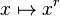$x \mapsto x^r$ are differentiable at 0 when$r \ge 1$. What's happening with the product rule example is that the two functions individually are not crossing that threshold, but when multiplied together, we get to the threshold.

The chain rule example can be thought of as restitution, or more descriptively, "the good washes out the bad". To understand this, it's worth noting that power functions$x \mapsto x^r$ are differentiable at 0 when$r \ge 1$. In the chain rule example,$f$ is good enough (high enough exponent) to wash out the "bad" exponent of$g$ and bring us to the threshold.

### What is really going on?

Given the subtle differences between the mechanisms for each of the examples, we may ask if there is any common thread to them. The underlying idea behind all of them is that, by combining functions in various ways, we can overcome the challenges that cause them to not be differentiable. The method needed can differ based on the type of operation (addition, multiplication, composition). Thus, the newly constructed function (sum, product, composite) may be differentiable at points where one or both of the original functions were not.

Another way of thinking about this is that combining functions through addition, multiplication, and composition is not reversible. So we "lose" information about individual functions when we combine them. With care, it is possible to specifically lose non-differentiability at specific points; basically, to smooth out the non-smooth behaviors.